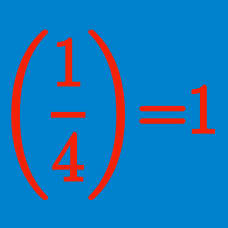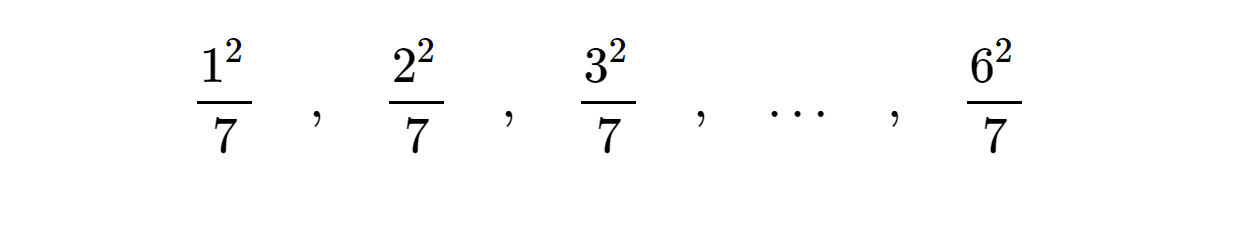Number Theory

#### Concept QuizzesIs 5 a quadratic residue modulo 7?

Which of these is the set of non-quadratic residues modulo $$11$$?

Which of these is the set of quadratic residues modulo $$15$$?

Find the sum of all positive integers $$x<22$$ such that $$x^2 \equiv 17 \pmod {22}$$.

Is $$15$$ a quadratic residue modulo $$17$$?

×